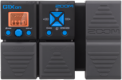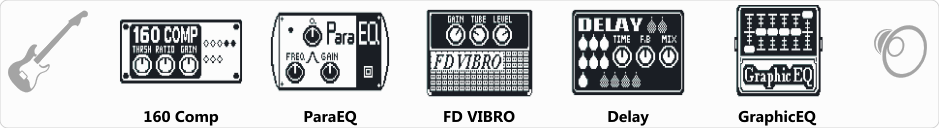# KL The Wall

Discussion in 'Zoom G1on/G1Xon' started by Kovács László, Jun 11, 2018.

1. KL The WallDevice: Zoom G1Xon
Firmware: 1.21

Name on device: The Wall
Optimized for: Phones/Speaker

Effects chain:LOUD! Use pedal for output volume!

Effect: "160 Comp" (Dynamics / Filter), active - "yes"
"THRSH" = -37
"Ratio" = 3.6
"Gain" = 9
"Knee" = Hard
"Level" = 120

Effect: "ParaEQ" (Dynamics / Filter), active - "yes"
"Freq1" = 100Hz
"Q1" = 1
"Gain1" = 8
"Freq2" = 2.5kHz
"Q2" = 0.5
"Gain2" = 11
"Level" = 70

Effect: "FD VIBRO" (Amp simulators), active - "yes"
"Gain" = 16
"Tube" = 0
"Level" = 150
"Trebl" = 21
"Middl" = 59
"Bass" = 76
"Prese" = 0
"CAB" = ALIEN 4x12
"OUT" = LINE

Effect: "Delay" (Delay / Reverb), active - "yes"
"Time" = 420
"F.B" = 27
"Mix" = 39
"HiDMP" = 10
"P-P" = MONO
"Level" = 100
"Tail" = Off

Effect: "GraphicEQ" (Dynamics / Filter), active - "yes"
"160Hz" = -4
"400Hz" = 1
"800Hz" = 4
"3.2kHz" = 6
"6.4kHz" = -5
"12kHz" = -2
"Level" = 100

Patch Volume: 100

Note: This is a patch file, you will need to download and install the ToneLib software to use the patch.

#### Attached Files:

• ###### KL_The_Wall.g1xon
File size:
424 bytes
Views:
305
tuncowsky and BA Gorimus like this.